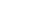[ back to Tom 7 Radar ]

w
e
b

l
i
n
k
The Quartic Formula (10 Feb 2005 at 17:55)
Man, did you ever want to solve an equation of the form x4 + ax3 + bx2 + cx + d = 0? Yeah? Well, no problem---just use this handy formula!

(Don't forget to scroll right...)c
o
m
m
e
n
t
jcreed (wittgenstein.wv.cc.cmu.edu) – 02.10.05 18:57:13
How convenient!
c
o
m
m
e
n
t
Quincunx (cpe-24-25-175-211.maine.res.rr.com) – 02.18.05 12:14:48
Oh... it's *plus* 27a²d, not minus... confound it, now I'll have to restart the entire calculation.
c
o
m
m
e
n
t
Pieter (pcp09645895pcs.mnhwkn01.nj.comcast.net) – 04.19.05 15:21:22
OMFG IM AM GOIGN TO KILL MYSELF
c
o
m
m
e
n
t
Tom 7 (gs82.sp.cs.cmu.edu) – 04.19.05 16:37:54
Don't do it!
c
o
m
m
e
n
t
Anonymous (24.127.51.37) – 05.27.05 18:53:43
How nerdy does your life need to be to need to use that in every day life? (yours truly)

I had to find this formula for my advanced calculus class, I typed it on equation editor. Forgot the -b/4a. HAD TO START THE WHOLE THING OVER!!!
c
o
m
m
e
n
t
mathwiz (68.145.162.118) – 05.28.05 16:02:13
thats quite a formula. i'll have fun memorizing it
c
o
m
m
e
n
t
Hot Tuna (212.219.204.66) – 10.10.05 11:02:24
Damn it! the formula wont show when I go to the site. Refresh doesn't work. I blame it on the trinity system.

Zounds! looks like I must search even more for a ridiculously long formula which can't solve ax^4 + bx^3 + cx^2 + dx + e. What if a<>1 ?
c
o
m
m
e
n
t
Tom 7 (gs82.sp.cs.cmu.edu) – 10.10.05 12:44:59
If a isn't 1, divide the whole polynomial by a. (If it's 0, use the cubic formula!)
c
o
m
m
e
n
t
Quintic? (d192-24-84-155.try.wideopenwest.com) – 05.13.06 13:22:54
anybody got ideas about quintic formula for my doctorate?
c
o
m
m
e
n
t
Anonymous (wsip-68-228-0-128.br.br.cox.net) – 06.13.06 20:31:56
no such formula exists, or can exist, without augmentation to elementary algebra. in any case, you're about half a century too late for this boat. no phd for you.
c
o
m
m
e
n
t
Anonymous (argus.hamiltondist.k12.wi.us) – 04.08.08 16:55:29
I'm trying to copy and paste to word so I can show my friends, but it's not working!!! the font gets too small when I down-size it!
c
o
m
m
e
n
t
Anonymous (argus.hamiltondist.k12.wi.us) – 04.08.08 16:59:12
Who likes cheesecake? Just go over to Mr. Akin's house tonight! We're making a poster w/ this formula on it for him!
c
o
m
m
e
n
t
Tom 7 (h-66-167-9-191.phlapafg.dynamic.covad.net) – 04.08.08 19:22:24
Where's the cheesecake though?
c
o
m
m
e
n
t
Chazthegreat (c-75-69-94-121.hsd1.nh.comcast.net) – 01.16.10 21:26:46
There's an error in the formula for r4. It's easy to see what they meant though. When you first load the page and scroll down just enough to see the formula for r4, most of the other equations are taken up by an expression of something over something. 2^(1/3)(b^2-3ac+12d) is the numerator. But that expression of something over something is not in the r4 formula. Where that expression is supposed to start, instead you see a smaller expression of something over nothing! But notice this: the numerators for both the expression of something over something, and for the expression of something over nothing, are the same. I said what the numerator is earlier. The expression following the expression of something over nothing starts with 3(2b^3-9abc... . This is the denominator of the expression of something over something.

The real formula for r4 is instead of having the expression of something over nothing, NEXT TO the expression that is the denominator of the expression of something over something, you have numerator of the expression of something over nothing OVER the expression that is the denominator of the expression of something over something.
c
o
m
m
e
n
t
Isn't there a formula with the x^4 coefficient (ax^4 +bx^3+cx^2+dx+e=0)?
c
o
m
m
e
n
t
Anonymous (host117-26-dynamic.33-79-r.retail.telecomitalia.it) – 06.02.10 13:48:25
3
c
o
m
m
e
n
t
Anonymous (hw-esr1-208-102-38-239.fuse.net) – 03.07.11 17:00:57
For anonymous at 01.17.10 14:59:39

If you want a formula for ax^4+bx^3+cx^2+dx+e=0, just divide it through by a:
x^4+(b/a)x^3+(c/a)x^2+(d/a)x+e=0p
o
s
t

a

c
o
m
m
e
n
tPlease enter the code, unless you are spammer!!